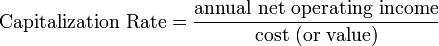# Difference Between Cap Rate and Discount Rate

By propertymetrics

What is the difference between a cap rate and a discount rate? Because these concepts are often confused, this article will discuss the difference between a capitalization rate and a discount rate in commercial real estate, and leave you with a clear understanding of the two concepts.

First, let’s go over a couple of definitions, and then we’ll dive into a specific example.

## Cap Rate

The capitalization rate, often just called the cap rate, is the ratio of Net Operating Income (NOI) to property asset value. So, for example, if a property was listed for \$1,000,000 and generated an NOI of \$100,000, then the cap rate would be \$100,000/\$1,000,000, or 10%.What is a cap rate in commercial real estate useful for? Because individual properties differ greatly in size and magnitude, it’s helpful to talk about property prices and values in a common language. Thinking of property value per dollar of current net income achieves this objective.

The cap rate is simply a measure that quantifies property value per dollar of current net income. Another way to think about the cap rate is that it’s the inverse of the popular price/earnings multiple used in the stock markets.

## Discount Rate

The discount rate is the rate used in a discounted cash flow analysis to compute present values.

When solving for the future value of money set aside today, we compound our investment at a particular rate of interest. When solving for the present value, the problem is one of discounting, rather than growing, and the required expected return acts as the discount rate. In other words, discounting is merely the inverse of growing.

What is the discount rate used for in commercial real estate analysis? In commercial real estate the discount rate is used in a discounted cash flow analysis to compute a net present value. Typically, the investor’s required rate of return is used as a discount rate, or in the case of an institutional investor, the weighted average cost of capital. This ensures that the initial investment made in a property achieves the investor’s return objectives, given the projected cash flows of the property. The intuition behind IRR and NPV is that it allows us to determine how much an investor should pay for a property, given his required rate of return, or discount rate.

## Cap Rate vs Discount Rate

So, back to the original question – what’s the difference between the cap rate versus the discount rate? The cap rate allows us to value a property based on a single year’s NOI. So, if a property had an NOI of \$80,000 and we thought it should trade at an 8% cap rate, then we could estimate its value at \$1,000,000.

The discount rate, on the other hand, is the investor’s required rate of return. The discount rate is used to discount future cash flows back to the present to determine value and account’s for all years in the holding period, not just a single year like the cap rate.

If a property’s cash flows are expected to increase or decrease over the holding period, then the cap rate will be a misleading performance indicator. Consider the following two investment alternatives:Both properties have a cap rate of 10% based on the NOI in year 1. But clearly the cash flows are better for Building B and it therefore provides a higher rate of return. The exact rate of return can be quantified using the Internal Rate of Return (IRR). Also, assuming equal risk, any rational investor should be willing to pay more for Building B because its future cash flows are expected to grow more than Building A’s. But how much more could you pay for Building B while still achieving your required return?

By completing a multiyear discounted cash flow analysis we could quantify exactly how much we can pay for this property with a Net Present Value (NPV), given an investor’s discount rate. The cap rate, on the other hand, will not be able to answer this question for us. In short, while the cap rate and the discount rate may appear similar, they are two different things used for different purposes.